Length

- You can measure how long things are, or how tall, or how far apart they are. Those are all examples of length measurements.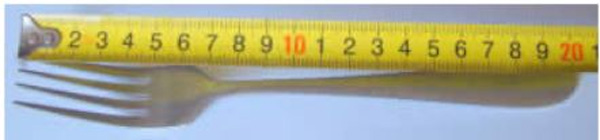Example: This fork is 20 centimeters long.

The units of length that are commonly used include:

• Millimeter (mm)
• Centimeter (cm)
• Decimetre (dm)
• Metre (m)
• Decametre (Dm)
• Hectometre (Hm)
• Kilometer (km)

meter is equal to 100 centimeters.

1 metre = 100 centimetres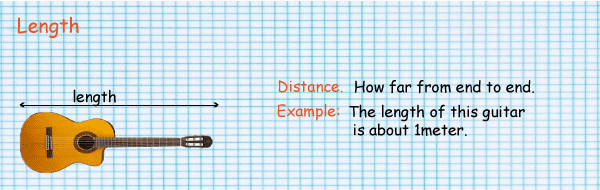- The length of this guitar is about 1 meter

- Meters might be used to measure the length of a house, or the size of a playground.

- And because a centimetre is 10 millimetres:

- 1 metre = 1000 millimetres

- A kilometre is equal to 1000 metres.

- When you need to get from one place to another, you measure the distance using kilometres.

The distance from one city to another or how far a plane travels would be measured using kilometres.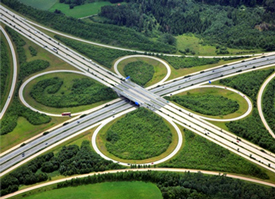Conversion of units can easily be done using the conversion below that is in form of a staircase.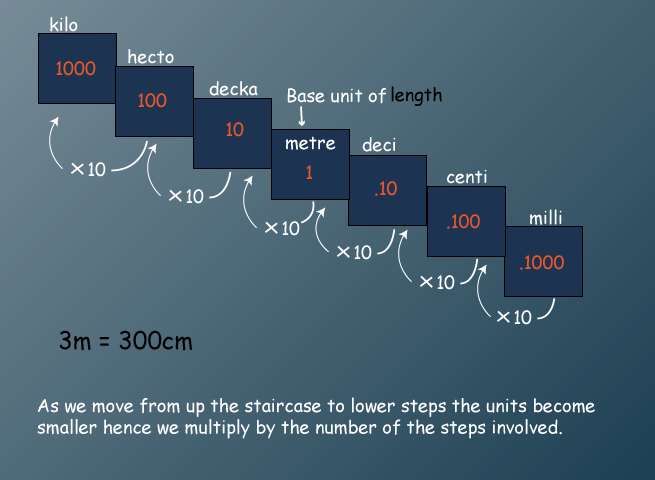#### Example

Tolome used a piece of string 8 `1/2` m long to support tomato plants in the garden.

The string was cut into pieces of 28 cm long. How many pieces were obtained?

Find also the length of the piece that remained to the nearest centimetre.

#### Solution

1 m = 100 cm

8.5m = ?

8.5 x 100 cm = 850 cm

one piece is 28 cm long

=850/28

=30 rem 10

Therefore: The Number of pieces = 30

Length of remaining piece = 10 cm.

### Units of Length

Recall that the most common metric units of length used are the kilometre (km), the metre (m), the centimetre (cm) and the millimetre (mm).

These units of length are related as follows:

10 mm = 1 cm

100 cm = 1 m

1000 m = 1 km

To convert length from a larger unit into a smaller unit, multiply by the relevant power of 10.

To convert length from a smaller unit into a larger unit, divide by the relevant power of 10.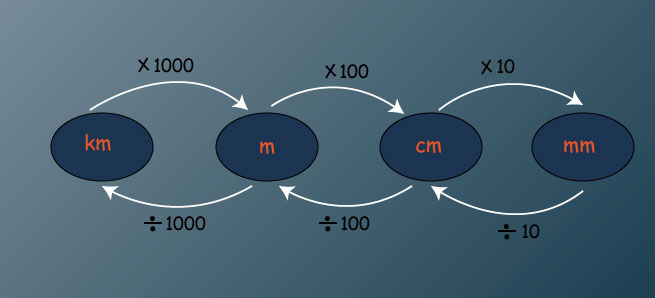a.  6 m to mm

b.  4 km to cm

c.  3 km to mm

#### Convert the following measurements to the units indicated

a.  62.8 cm to mm

b.  48.7 m to cm

c.  8.26 m to mm

d.  4.75 km to mm

##### Solution

a. 62.8 cm = 62.8 x10 mm                      1 cm = 10mm

= 628 mm

b. 48.7 m = 48.7 x100 cm                        1 m = 100cm

= 4870 cm

c. 8.26 m = 8.26 x 100 cm                          1m = 100 cm

= 8.26 cm

= 826 x 10 mm

= 8260 mm

d. 4.75 km = 4.75 x 1000 m                      1 km= 1000 m

= 4750 m                                  1m = 100 cm

= 4750 x 100 cm                      1 cm = 10 mm

= 475 000 cm

= 475 000 x 10 mm

= 4750 000 mm

#### Convert the following into metres

a) 120 cm 10 mm

b) 2 Km 5 Hm

#### Solution

a) 100 cm = 1 m = 1000 mm

120 cm =  `120/100` m = 1.2 m

10 mm = `10/1000` m = 0.01 m

120 cm 10 mm = (1.2 + 0.01) m

= 1.21 m

b) 1 Km = 1000 m , 1 Hm = 100 m

2 Km =2 x 1000 m = 2000 m

5 Hm = 5 x 100 m = 500 m

2 Km 5 Hm = ( 2000+500) m

= 2500 m

## KCPE Topical QUestions

Class 7

### Length and its operations

1. In a trapezium ABCD, AB is parallel to DC. BC is perpendicular to AB and DC. AB = 4 cm and BC = 5 cm. The area of trapezium is 100 cm2. What is the length of DC?

A. 26 cm     B. 6 cm     C. 20 cm       D. 19 cm

2. Konchella wants to construct a timber wall 6 m long and 2.25 m high. Timber is to be placed vertically as shown in the diagram below.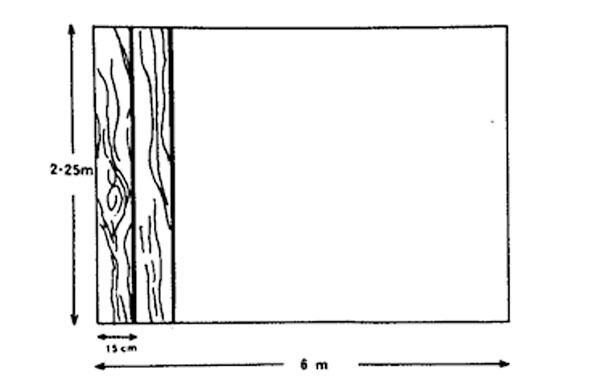How many pieces of timber each of 4.5 m long and 15 cm wide would he require to construct the wall?

A. 40       B. 20      C. 80       D. 4

3. Kirimi’s rectangular plot of land measures 100 m by 50 m. he wanted to fence around it using 3 strands of wire. He had 3 rolls of wire each measuring 350 m. What is the length of the wore that will remain after he has fenced the plot?

A. 50 m    B. 150 m     C. 750 m       D. 900 m

4. The length of a rectangular sheet of paper is 3 cm more than its breadth. The sheet of paper has a perimeter of 42 cm. What is its length?

A. 9 cm     B. 12 cm    C. 19.5 cm      D. 22.5 cm

5. The diagram below represents Kaari’s vegetable garden. Kaari intends to fence the garden using four strands of wire.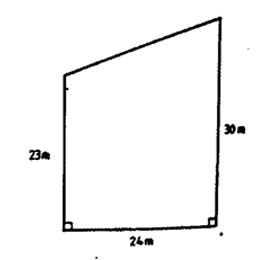What length of wire, in meters, does she require?

A. 408     B. 404     C. 308       D. 102

6. The area of the trapezium EFGH below is 3000 cm2. The length of EH is 80 cm and that of FG is 120 cm. EH is parallel to FG and HG is perpendicular to EH and FG.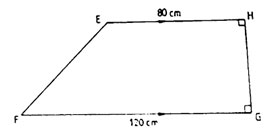What is the length of GH?

A. 50 cm        B. 30 cm        C. 25 cm        D. 15 cm